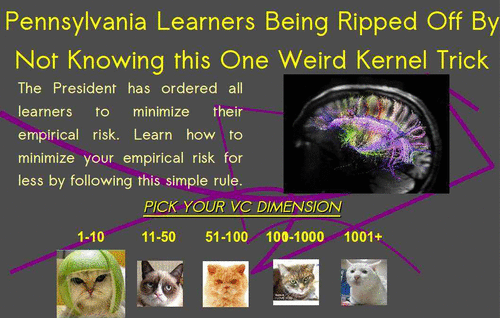# (Reproducing) kernel tricksWARNING: This is very old. If I were to write it now, I would write it differently, and specifically more pedagogically.

Kernel in the sense of the “kernel trick”. Not to be confused with smoothing-type convolution kernels, nor the dozens of related-but-slightly-different clashing definitions of kernel; those can have their own respective pages. Corollary: If you do not know what to name something, call it a kernel.

We are concerned with a particular flavour of kernel in Hilbert spaces, specifically reproducing or Mercer kernels . The associated function space is a reproducing Kernel Hilbert Space, which is hereafter an RKHS.

Kernel tricks comprise the application of Mercer kernels in Machine Learning. The “trick” part is that many machine learning algorithms operate on inner products. Or can be rewritten to work that way. Such algorithms permit one to swap out a boring classic Euclidean definition of that inner product in favour of a fancy RKHS one. The classic machine learning pitch for trying such a stunt is something like “upgrade your old boring linear algebra on finite (usually low-) dimensional spaces to sexy algebra on potentially-infinite-dimensional feature spaces, which still has a low-dimensional representation.” Or, if you’d like, “apply certain statistical learning methods based on things with an obvious finite vector space representation ($$\mathbb{R}^n$$) to things without one (Sentences, piano-rolls, $$\mathcal{C}^d_\ell$$).”

Mini history: The oft-cited origins of all the reproducing kernel stuff are . It took a while to percolate into random function theory as covariance functions. Thence the idea arrived in statistical inference and signal processing , and now it is ubiquitous.

Practically, kernel methods have problems with scalability to large data sets. To apply any such method you need to keep a full Gram matrix of inner products between every data point, which needs you to know, for $$N$$ data points, $$N(N-1)/2$$ entries of a symmetric matrix. If you need to invert that matrix the cost is $$\mathcal{O}(N^3)$$, which means you need fancy tricks to handle large $$N$$. Fancy tricks depend on what the actual model is, but include Sparse GPs, random-projection inversions, Markov approximations and presumably many more

I’m especially interested in the application of such tricks in

1. kernel regression
2. wide random NNs
3. Nonparametric kernel independence tests
4. Efficient kernel pre-image approximation
5. Connection between kernel PCA and clustering Turns out not all those applications are interesting to me.

## IntroductionsFeature space

There are many primers on Mercer kernels and their connection to ML. Kenneth Tay’s intro is punchy. See , which grinds out many connections with learning theory, or , which is more narrowly focussed on just the Mercer-kernel part which emphasises topological and geometric properties of the spaces, or for an approximation-theory perspective which does not especially concern itself with stochastic processes. I also seem to have bookmarked the following introductions .

Alex Smola (who with, Bernhard Schölkopf) has his name on an intimidating proportion of publications in this area, also has all his publications online.

## Non-scalar-valued “kernels”

Extending the usual inner-product framing, Operator-valued kernels, , generalise to $$k:\mathcal{X}\times \mathcal{X}\mapsto \mathcal{L}(H_Y)$$, as seen in multi-task learning.

## Tools

### KeOps

File under least squares, autodiff, gps, pytorch.

The KeOps library lets you compute reductions of large arrays whose entries are given by a mathematical formula or a neural network. It combines efficient C++ routines with an automatic differentiation engine and can be used with Python (NumPy, PyTorch), Matlab and R.

It is perfectly suited to the computation of kernel matrix-vector products, K-nearest neighbors queries, N-body interactions, point cloud convolutions and the associated gradients. Crucially, it performs well even when the corresponding kernel or distance matrices do not fit into the RAM or GPU memory. Compared with a PyTorch GPU baseline, KeOps provides a x10-x100 speed-up on a wide range of geometric applications, from kernel methods to geometric deep learning.

### Falkon

A Python library for large-scale kernel methods, with optional (multi-)GPU acceleration.

The library currently includes two solvers: one for approximate kernel ridge regression Rudi, Carratino, and Rosasco (2017) which is extremely fast, and one for kernel logistic regression Marteau-Ferey, Bach, and Rudi (2019) which trades off lower speed for better accuracy on binary classification problems.

The main features of Falkon are:

• Full multi-GPU support - All compute-intensive parts of the algorithms are multi-GPU capable.
• Extreme scalability - Unlike other kernel solvers, we keep memory usage in check. We have tested the library with datasets of billions of points.
• Sparse data support
• Scikit-learn integration - Our estimators follow the scikit-learn API

### No comments yet. Why not leave one?

GitHub-flavored Markdown & a sane subset of HTML is supported.